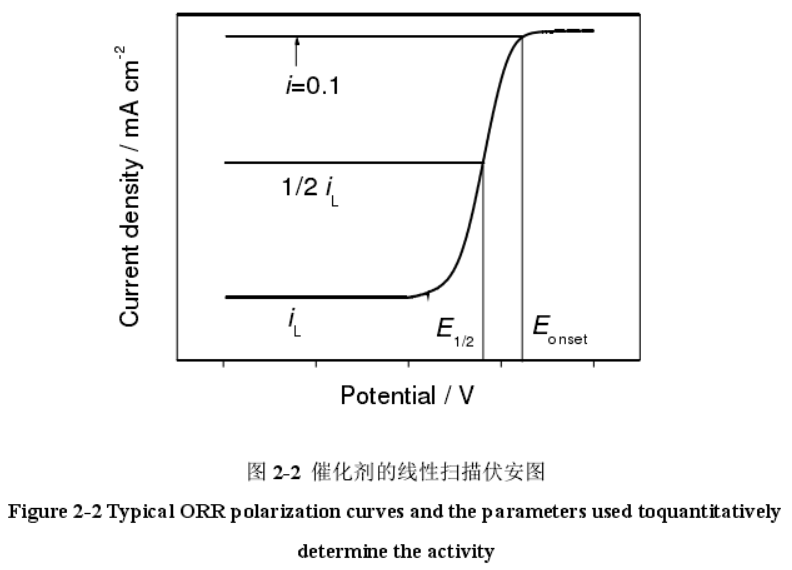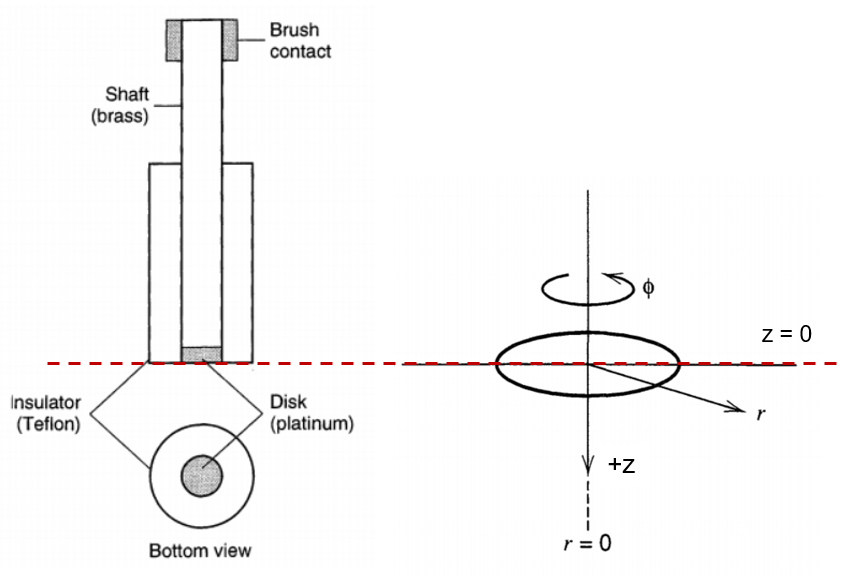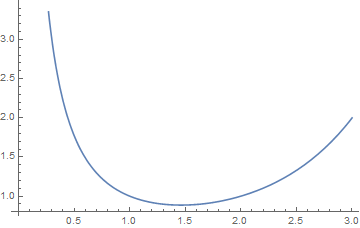0%

# 写在前面Ref: 周学俊. M-Nx/C类非贵金属氧还原催化材料的构筑及其电化学性能研究[D]. 东华大学, 2016.

K-L方程实际上建立了极限扩散电流$i_l$与旋转圆盘转速等物理因素之间的关系。通过不同转速下测试极限扩散电流，便可以拟合出关键的反应动力学参数——转移电子数。那么极限扩散电流到底是不是材料的本征性质呢？这个问题没有想象的那么简单，笔者首先介绍了K-L理论后我们再作判断不迟。

# RDE下液流层的流体力学描述Ref: Bard et al., Electrochemical Methods: Fundamentals and Applications; wiley New York, 1980; Vol. 2.

• 圆盘表面液体流速满足$v_r=0, v_phi=0, v_y=\omega r$.
• 本体溶液液体流速满足$v_r=0, v_phi=0, v_y=-U_0$.

Ref: Real-Ramirez, C. A., & Gonzalez-Trejo, J. I. (2011). Hydrodynamic Analysis of Electrochemical Cells. In Computational Simulations and Applications. IntechOpen.

von Karman在1921年提出以独立无量纲变量来描述各方向速度值，可以满足N-S方程的限制条件，即

Ref: von Kármán, T. (1921). Über laminare und turbulente reibung. Zeitschrift für Angewandte Mathematik und Mechanik, 1, 486.

Chochran在1934年提出使用无穷级数方法近似表示$F, G, H$三个函数。在离电极表面较近处，即$z$较小时，$\gamma << 1$，此时各方向速度值可表示为

Ref: Cochran, W. G. (1934). The flow due to a rotating disc. Proceedings of the Cambridge Philosophical Society, 30, 365-375.

# RDE法向的物质对流及扩散

$$v_z (\frac{\partial C_o}{\partial z}) = D_o \frac{\partial^2 C_o}{\partial z^2}$$

## 极限电流情形$$i_l = 0.62 nFAD^{2/3} \omega^{1/2} \mu^{-1/6} C_o^*$$

## 非极限电流情形

$$\frac{1}{i} = \frac{1}{i_k} + \frac{1}{i_l} = \frac{1}{i_k} + \frac{1}{0.62 nFAD^{2/3} \omega^{1/2} \mu^{-1/6} C_o^*}$$# 写在后面

• 如果电极的表面物理化学性质发生变化（也即电极经过表面修饰），那么反应的转移电子数必定会发生变化，从而反映在K-L方程拟合直线的斜率截距上。
• 在正确的表征方法下，极限扩散电流是材料的本征性质（活性位点催化选择反应路径的性质）。
• 负载量可能会通过活性位点暴露的角度对极限扩散电流产生影响。但在一定范围内，其影响可能是不大的。
• 在ORR测试中，需要通过K-L方程计算不同偏压下的转移电子数。这时候一定要注意各数值的量纲问题！在转速使用“rpm”（Revolutions Per Minute，圈/分）时，常数取“0.2”， 即使用公式：$i_l = 0.2 nFAD_o^{2/3} \omega^{1/2} \mu^{-1/6} C_o^*$；在转速使用“rad/s”（Radian Per Second，弧度/秒）时，常数取“0.62”， 即使用公式：$i_l = 0.62 nFAD_o^{2/3} \omega^{1/2} \mu^{-1/6} C_o^*$（如果纵坐标已经取电流密度，记得将等式右边的A略掉）。

• ORR计算常用物理常数：法拉第常数$F=96485 C mol^{-1}$，氧气在电解液中的饱和浓度$C_o = 1.2 \times 10^{-3} mol L^{-1}$，氧气在KOH溶液中的扩散系数$D_o = 1.9 \times 10^{-5} cm s^{-1}$，电解液粘度$\mu = 0.01 cm^2 s^{-1}$.

# 参考文献

 Bard, A. J., Faulkner, L. R., Leddy, J., & Zoski, C. G. (1980). Electrochemical methods: fundamentals and applications (Vol. 2). New York: wiley.

 Real-Ramirez, C. A., & Gonzalez-Trejo, J. I. (2011). Hydrodynamic Analysis of Electrochemical Cells. In Computational Simulations and Applications. IntechOpen.

 von Kármán, T. (1921). Über laminare und turbulente reibung. Zeitschrift für Angewandte Mathematik und Mechanik, 1, 486.

 Cochran, W. G. (1934). The flow due to a rotating disc. Proceedings of the Cambridge Philosophical Society, 30, 365-375.

 Han S, Hu X, Wang J, et al. Novel Route to Fe‐Based Cathode as an Efficient Bifunctional Catalysts for Rechargeable Zn–Air Battery[J]. Advanced Energy Materials, 2018, 8(22): 1800955.

 Liang Y, Li Y, Wang H, et al. Co 3 O 4 nanocrystals on graphene as a synergistic catalyst for oxygen reduction reaction[J]. Nature materials, 2011, 10(10): 780-786.